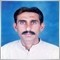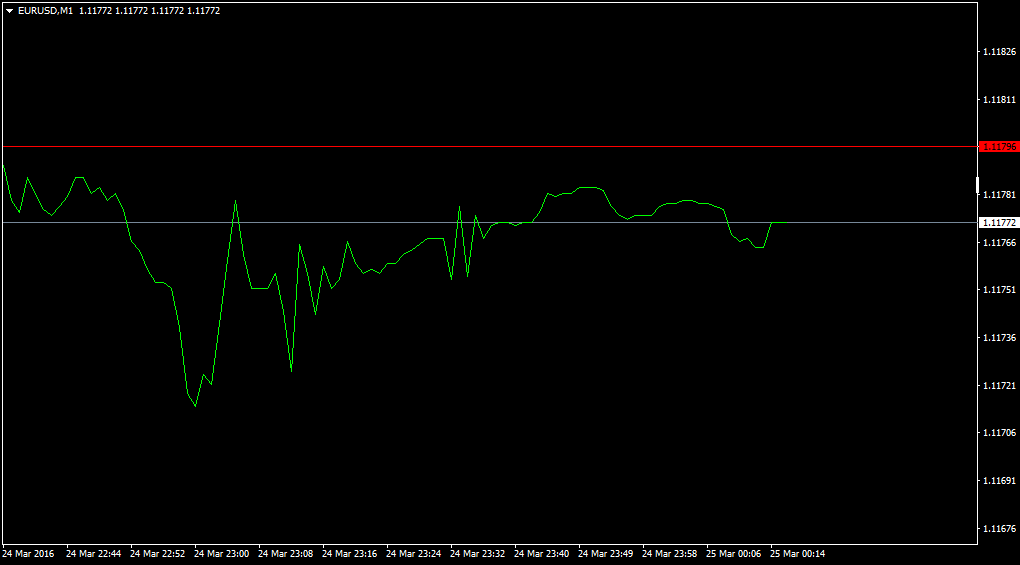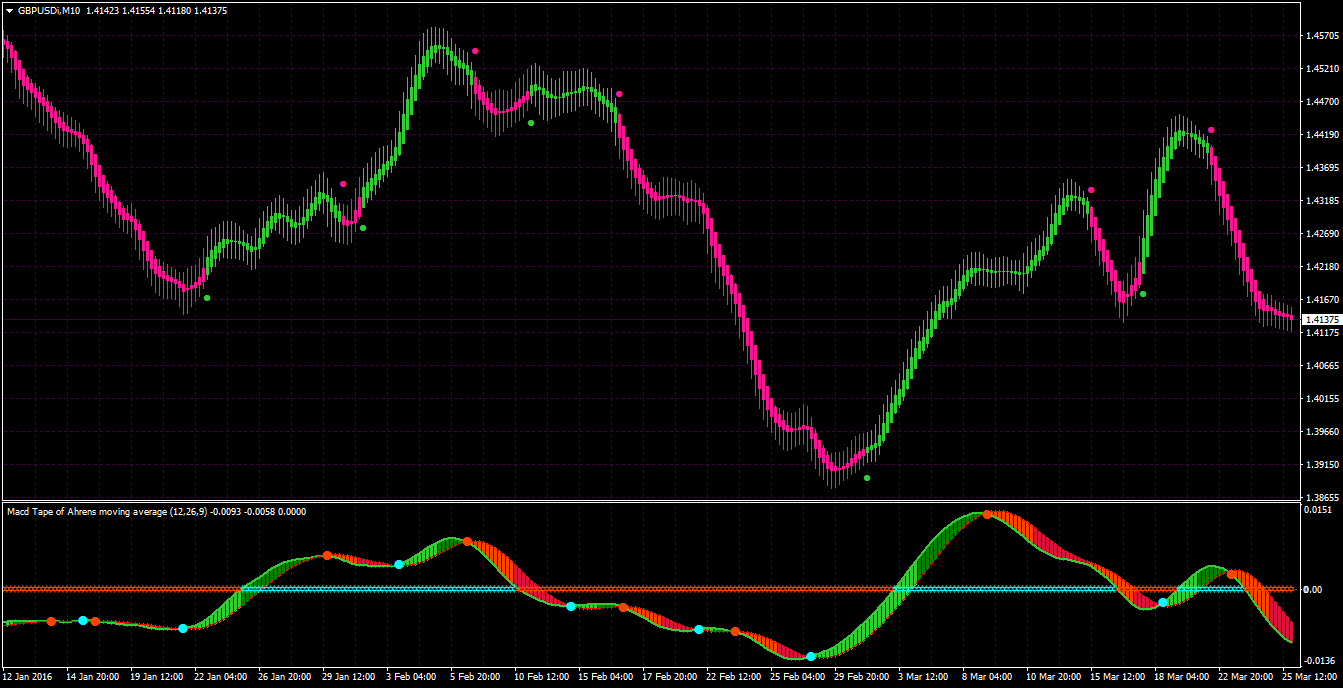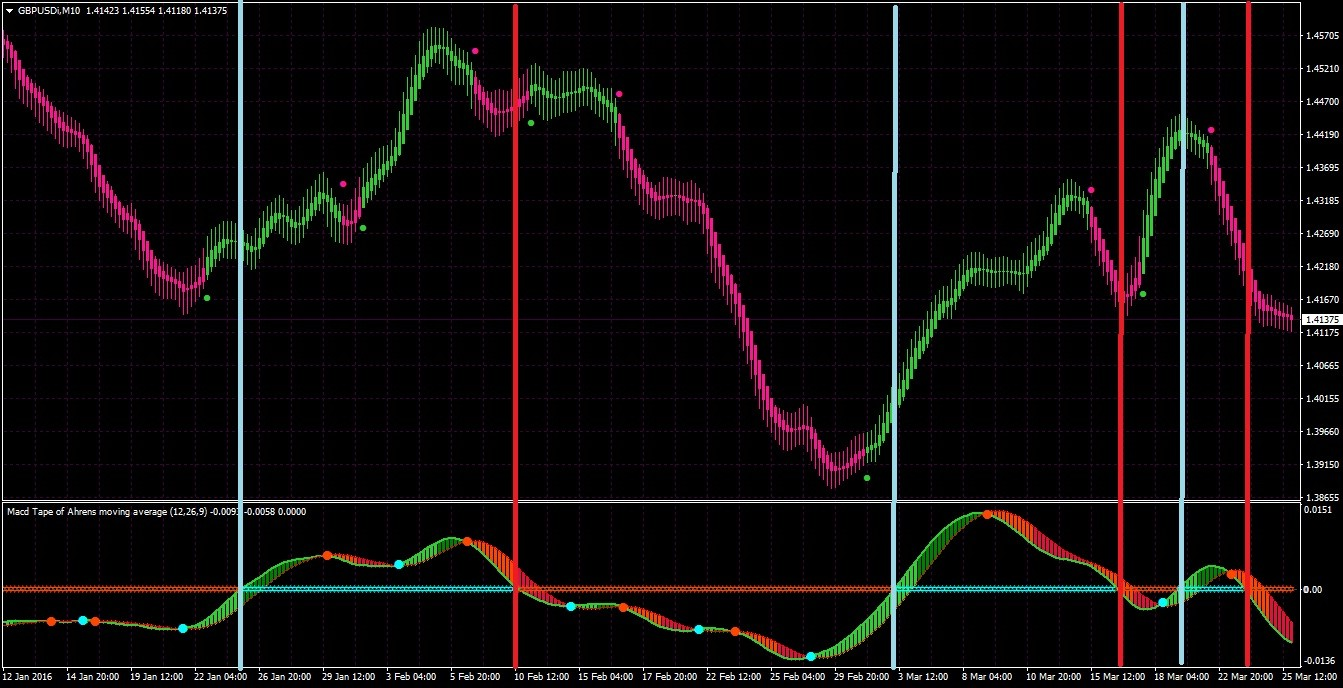Elite indicators :) - page 119034

Files:4535

mandagozu81

That "prices" can be used only in specific indicators that are using multiple prices in some cases. For example stochastic and some step indicators allow that. But others simple can not use more than one price and for that reason they can not use those price combinations

one question regarding price calculation,at any chart with any tf and pair...... bars and or candles are calculated with any or a combination of from 4 price type values (open,close,low and high)...my question is, how line chart is calculated,if with close price value only or (open+close+low+high/4) value ?

regardsFiles:151508

mntiwana:

one question regarding price calculation,at any chart with any tf and pair...... bars and or candles are calculated with any or a combination of from 4 price type values (open,close,low and high)...my question is, how line chart is calculated,if with close price value only or (open+close+low+high/4) value ?

regardsmntiwana

The difference is that, for example stochastic, adx and some more use 3 prices/value in calculations, some step algorithms allow 2 prices//value, while, for example, average simply uses one price/value - there is no place where to use more than one price value. So it depends on what is calculated how much prices/values can be used. So it is not a matter of price/value, but a matter how many of those prices/values can be used, at the same time - not as some average value (like the (open+close+low+high/4)), in calculation4535

mntiwana

The difference is that, for example stochastic, adx and some more use 3 prices/value in calculations, some step algorithms allow 2 prices//value, while, for example, average simply uses one price/value - there is no place where to use more than one price value. So it depends on what is calculated how much prices/values can be used. So it is not a matter of price/value, but a matter how many of those prices/values can be used, at the same time - not as some average value (like the (open+close+low+high/4)), in calculation

sorry i was unable to exact explain my question.

thanks for explained reply,what you described,it is about when we use some indicator,i want know when no indicator applied,how the line graph form/move at chart as price line......when a simple/empty (without any indicator) chart is in bar and candle mode,it forming bars and candles showing (open,close.high,low),so when we apply line graph mode,what i observed,it form line from previous close to current close and so on,close to close....so my curiosity is about line graph chart,if line forms with close price,in other words line graph charts forms with close price only,so it is a price line of close value price.

regards151508

mntiwana:

sorry i was unable to exact explain my question.

thanks for explained reply,what you described,it is about when we use some indicator,i want know when no indicator applied,how the line graph form/move at chart as price line......when a simple/empty (without any indicator) chart is in bar and candle mode,it forming bars and candles showing (open,close.high,low),so when we apply line graph mode,what i observed,it form line from previous close to current close and so on,close to close....so my curiosity is about line graph chart,if line forms with close price,in other words line graph charts forms with close price only,so it is a price line of close value price.

regards

mntiwana

Yes, metatrader line graph uses only close prices9497

This is a macd averages tape, the indicator will place a dot on the macd line when the macd and signal cross, and will color the zero line with another type of dot for zero line crosses and will stay in that mode until a zero cross in the other direction happens. The tape bars are colored when the difference in the macd and signal is increasing(gaining momentum) and if the difference is decreasing(momentum slowing) they change color. The macd can be calculated using the latest averages with the double smoothing method.

Macd averages tape.ex4Files:4535

mrtools:
This is a macd averages tape, the indicator will place a dot on the macd line when the macd and signal cross, and will color the zero line with another type of dot for zero line crosses and will stay in that mode until a zero cross in the other direction happens. The tape bars are colored when the difference in the macd and signal is increasing(gaining momentum) and if the difference is decreasing(momentum slowing) they change color. The macd can be calculated using the latest averages with the double smoothing method.

Macd averages tape.ex4Dearest MRTOOLS

really a great and nice tool,much thanks for pretty work.

regards967

mrtools:
This is a macd averages tape, the indicator will place a dot on the macd line when the macd and signal cross, and will color the zero line with another type of dot for zero line crosses and will stay in that mode until a zero cross in the other direction happens. The tape bars are colored when the difference in the macd and signal is increasing(gaining momentum) and if the difference is decreasing(momentum slowing) they change color. The macd can be calculated using the latest averages with the double smoothing method.

Macd averages tape.ex4Hi Mrtools

It is very good visually helping idea, I suggest to add vertical lines instead dots or arrows when the zero line color are changed its modeMy regards151508

talaate:

Hi Mrtools

It is very good visually helping idea, I suggest to add vertical lines instead dots or arrows when the zero line color are changed its modeMy regards

Talaat E

Then the line would take 3 buffers instead of 2 (otherwise it would repaint). I believe that mrtools did it that way to make it as simple as possible for usage967

Talaat E

Then the line would take 3 buffers instead of 2 (otherwise it would repaint). I believe that mrtools did it that way to make it as simple as possible for usage